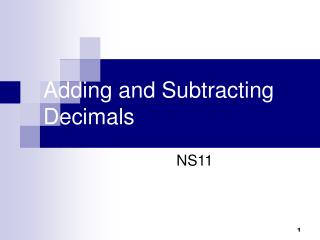DownloadDownload PresentationTélécharger la présentation- - - - - - - - - - - - - - - - - - - - - - - - - - - E N D - - - - - - - - - - - - - - - - - - - - - - - - - - -
##### Presentation Transcript

1. Recall that when we add or subtract money, we write the numbers so that the decimal points are vertically aligned. • This way we are sure to add digits with the same place value. • We insert the decimal point in the answer below the other decimal points, as shown above.

2. We use the same procedure to add or subtract any decimal numbers. • We keep the decimal points in line. • This way, we add or subtract digits with the same place value. • The decimal points stay in a straight line, as shown above.

3. Let’s practice! • Find the perimeter of the triangle. Units are in centimeters. • How do we find perimeter? • We keep the decimal points aligned in the problem so we can add digits with the same place value. • We add the digits column by column, just as we would add whole numbers or money.

4. Another Example: • The roof was 6.37 meters above the ground. The ladder could reach only 4.2 meters. The roof was how much higher than the ladder could reach? • We line up the decimal points vertically so that we subtract digits with the same place value. • We attach a zero to 4.2 so that there are no empty places in the problem. Then we subtract.

5. Add: 6.2+3+ 4.25 • To add digits with the same place value, we align decimal points. • In this problem the whole number 3 has the same place value as the 6 and the 4. • We place a decimal point to the right of the 3 and align decimal points. • We may fill empty decimal places with zeros if we wish.

6. For some subtraction problems we need to add decimal places to perform the subtraction. • If we subtract 0.23 from 0.4, we find there is an “empty” place in the problem. • We fill the empty place with a zero. Then we subtract.

7. Subtract: 0.4 – 0.231 • We set up the problem by lining up the decimal points, remembering to write the first number on top. • We fill empty places with zeros. • Then we subtract.

8. Subtract: 3 – 1.23 • This problem is similar to subtracting \$1.23 from \$3. • We place the decimal point to the right of the 3, fill the decimal places with zeros, and subtract.

9. 4.3 + 2 • 4.5 • 6.3 • 4.1 • 2.3

10. 4 + 1.3 + 0.6 • 2.3 • 5.3 • 5.9

11. 4.75 – 2 • 2.75 • 4.73 • 6.75 • 4.77

12. 0.3 – 0.15 • 0.25 • 0.15 • 0.12 • 0.18

13. 1 – 0.1 • 0.9 • 1.1 • 0

14. 2.5 – 1 • 2.4 • 2.6 • 1.6 • 1.4

15. 1 – 0.25 • 0.24 • 0.75 • 1.25 • 0.15

16. The end!